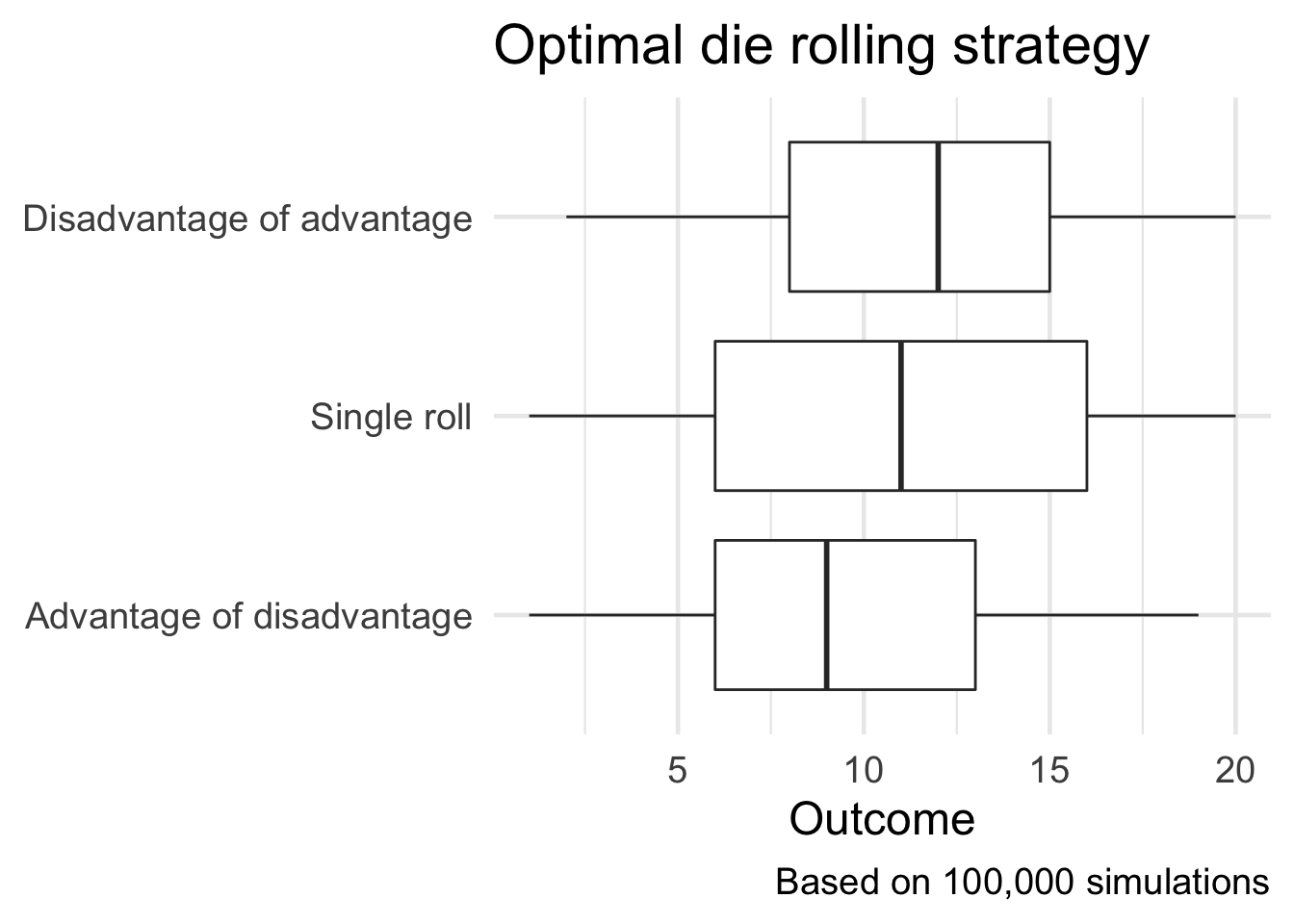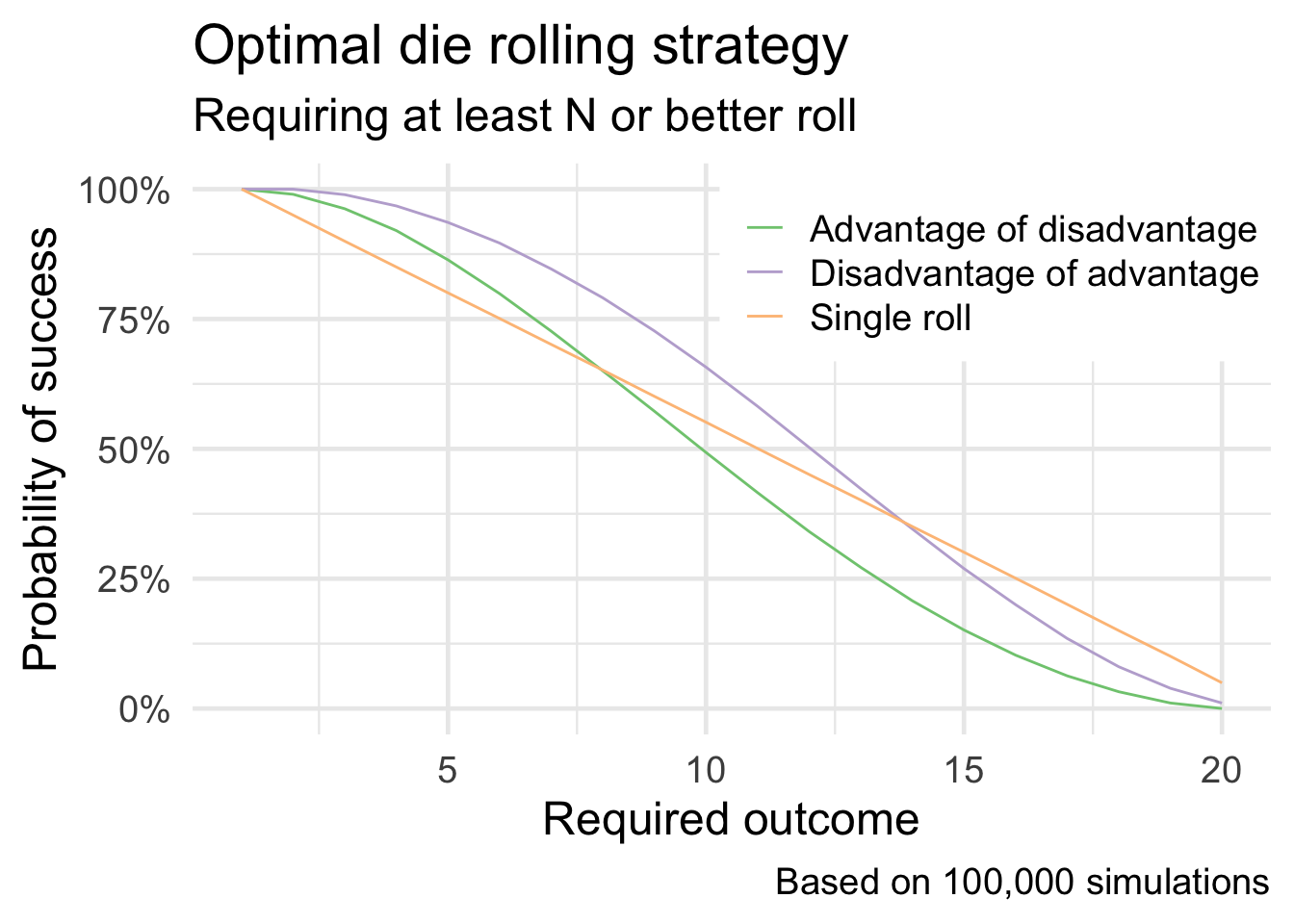I enjoyed the recent Riddler challenger from May 15th:

The fifth edition of Dungeons & Dragons introduced a system of “advantage and disadvantage.” When you roll a die “with advantage,” you roll the die twice and keep the higher result. Rolling “with disadvantage” is similar, except you keep the lower result instead. The rules further specify that when a player rolls with both advantage and disadvantage, they cancel out, and the player rolls a single die. Yawn!

Extra Credit: Instead of maximizing your expected roll, suppose you need to roll N or better with your 20-sided die. For each value of N, is it better to use advantage of disadvantage, disadvantage of advantage or rolling a single die?

To simulate this, I need to write a few functions to sample from a random number generator simulating the die rolls. I’m sure I could have written these more efficiently, but I can take advantage of purrr::map() and basic iterative operations to repeatedly simulate this process.

library(tidyverse)

## ── Attaching packages ─────────────────────────────────────── tidyverse 1.3.1 ──

## ✓ ggplot2 3.3.3     ✓ purrr   0.3.4
## ✓ tibble  3.1.1     ✓ dplyr   1.0.6
## ✓ tidyr   1.1.3     ✓ stringr 1.4.0
## ✓ readr   1.4.0     ✓ forcats 0.5.1

## ── Conflicts ────────────────────────────────────────── tidyverse_conflicts() ──

library(glue)

##
## Attaching package: 'glue'

## The following object is masked from 'package:dplyr':
##
##     collapse

set.seed(123)

theme_set(theme_minimal(base_size = 18))

# constants
n_sides <- 20
n_sim <- 1e05

advantage <- function(n_sides = 20) {
# roll twice, keep the higher value
roll <- sample.int(n = n_sides, size = 2)
roll <- roll[which.max(roll)]

return(roll)
}

disadvantage <- function(n_sides = 20) {
# roll twice, keep the lower value
roll <- sample.int(n = n_sides, size = 2)
roll <- roll[which.min(roll)]

return(roll)
}

adv_dis <- function(n_sides = 20) {
# roll twice with disadvantage and then keep the higher result
roll <- rerun(.n = 2, disadvantage()) %>%
as_vector()
roll <- roll[which.max(roll)]

return(roll)
}

dis_adv <- function(n_sides = 20) {
# roll twice with advantage and then keep the lower result
roll <- rerun(.n = 2, advantage()) %>%
as_vector()
roll <- roll[which.min(roll)]

return(roll)
}

# single roll
single_roll <- function(n_sizes = 20) {
# roll the N-sided die once
sample.int(n = n_sides, size = 1)
}


From here, it’s simply a matter of testing each strategy lots of times and averaging over the resulting outcomes.

# simulate a bunch of rolls
rolls <- tibble(
.id = 1:n_sim
) %>%
mutate(Advantage of disadvantage = map_dbl(.id, adv_dis),
Disadvantage of advantage = map_dbl(.id, dis_adv),
Single roll = map_dbl(.id, single_roll)) %>%
# collect outcomes into a tidy format
pivot_longer(cols = -.id, names_to = "type_roll", values_to = "outcome")

# expected value of each method
rolls %>%
group_by(type_roll) %>%
summarize(exp_val = mean(outcome)) %>%
knitr::kable(col.names = c("Strategy", "Expected value"))

Strategy Expected value
Single roll 10.512
ggplot(data = rolls, mapping = aes(x = fct_reorder(type_roll, outcome), y = outcome)) +
geom_boxplot() +
coord_flip() +
labs(
title = "Optimal die rolling strategy",
caption = glue("Based on {scales::comma(n_sim)} simulations"),
x = NULL,
y = "Outcome"
)As for the extra credit challenge, rather than averaging over all the outcomes I need to check for each possible value for $$N$$ whether the roll would have been successful or not.

# which is optimal method for needing to roll N or better
n_better <- function(req_out = 10, rolls_df) {
rolls_df %>%
mutate(success = outcome >= req_out)
}

rolls_by_n <- tibble(
n = 1:20
) %>%
mutate(results = map(n, n_better, rolls_df = rolls)) %>%
unnest(cols = c(results)) %>%
group_by(n, type_roll) %>%
summarize(success = mean(success))

## summarise() has grouped output by 'n'. You can override using the .groups argument.

ggplot(
data = rolls_by_n,
mapping = aes(x = n, y = success, color = type_roll)
) +
geom_line() +
scale_y_continuous(labels = scales::percent) +
scale_color_brewer(type = "qual") +
labs(
title = "Optimal die rolling strategy",
subtitle = "Requiring at least N or better roll",
caption = glue("Based on {scales::comma(n_sim)} simulations"),
x = "Required outcome",
y = "Probability of success",
color = NULL
) +
theme(
legend.position = c(.75, .83),
legend.background = element_rect(fill = "white", size = 0)
)Initially, advantage of disadvantage is the preferred strategy until $$N > 14$$, at which point the single roll strategy is optimal.

Posted on:
May 15, 2020
Length: